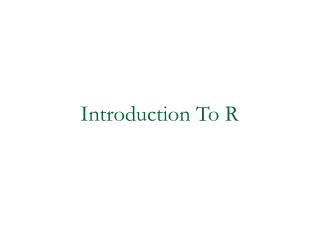# Introduction To R - PowerPoint PPT PresentationDownload PresentationIntroduction To R

Introduction To R
Download Presentation## Introduction To R

- - - - - - - - - - - - - - - - - - - - - - - - - - - E N D - - - - - - - - - - - - - - - - - - - - - - - - - - -
##### Presentation Transcript

1. Introduction To R

2. What is R? • The R statistical programming language is a free open source package based on the S language developed by Bell Labs. • The language is very powerful for writing programs. • Many statistical functions are already built in. • Contributed packages expand the functionality to cutting edge research. • Since it is a programming language, generating computer code to complete tasks is required.

3. Getting Started • Where to get R? • Go to www.r-project.org • Downloads: CRAN • Set your Mirror: Anyone in the USA is fine. • Select Windows 95 or later. • Select base. • Select R-2.4.1-win32.exe • The others are if you are a developer and wish to change the source code. • UNT course website for R: • http://www.unt.edu/rss/SPLUSclasslinks.html

4. Getting Started • The R GUI?

5. Getting Started • Opening a script. • This gives you a script window.

6. Getting Started • Basic assignment and operations. • Arithmetic Operations: • +, -, *, /, ^ are the standard arithmetic operators. • Matrix Arithmetic. • * is element wise multiplication • %*% is matrix multiplication • Assignment • To assign a value to a variable use “<-”

7. Getting Started • How to use help in R? • R has a very good help system built in. • If you know which function you want help with simply use ?_______ with the function in the blank. • Ex: ?hist. • If you don’t know which function to use, then use help.search(“_______”). • Ex: help.search(“histogram”).

8. Importing Data • How do we get data into R? • Remember we have no point and click… • First make sure your data is in an easy to read format such as CSV (Comma Separated Values). • Use code: • D <- read.table(“path”,sep=“,”,header=TRUE)

9. Working with data. • Accessing columns. • D has our data in it…. But you can’t see it directly. • To select a column use D\$column.

10. Working with data. • Subsetting data. • Use a logical operator to do this. • ==, >, <, <=, >=, <> are all logical operators. • Note that the “equals” logical operator is two = signs. • Example: • D[D\$Gender == “M”,] • This will return the rows of D where Gender is “M”. • Remember R is case sensitive! • This code does nothing to the original dataset. • D.M <- D[D\$Gender == “M”,] gives a dataset with the appropriate rows.

11. Basic Graphics • Histogram • hist(D\$wg)

12. Basic Graphics • Add a title… • The “main” statement will give the plot an overall heading. • hist(D\$wg , main=‘Weight Gain’)

13. Basic Graphics • Adding axis labels… • Use “xlab” and “ylab” to label the X and Y axes, respectively. • hist(D\$wg , main=‘Weight Gain’,xlab=‘Weight Gain’, ylab =‘Frequency’)

14. Basic Graphics • Changing colors… • Use the col statement. • ?colors will give you help on the colors. • Common colors may simply put in using the name. • hist(D\$wg, main=“Weight Gain”,xlab=“Weight Gain”, ylab =“Frequency”, col=“blue”)

15. Basic Graphics – Colors

16. Basic Plots • Box Plots • boxplot(D\$wg)

17. Boxplots • Change it! • boxplot(D\$wg,main='Weight Gain',ylab='Weight Gain (lbs)')

18. Box-Plots - Groupings • What if we want several box plots side by side to be able to compare them. • First Subset the Data into separate variables. • wg.m <- D[D\$Gender=="M",] • wg.f <- D[D\$Gender=="F",] • Then Create the box plot. • boxplot(wg.m\$wg,wg.f\$wg)

19. Boxplots – Groupings

20. Boxplots - Groupings boxplot(wg.m\$wg, wg.f\$wg, main='Weight Gain (lbs)', ylab='Weight Gain', names = c('Male','Female'))

21. Boxplot Groupings • Do it by shift • wg.7a <- D[D\$Shift=="7am",] • wg.8a <- D[D\$Shift=="8am",] • wg.9a <- D[D\$Shift=="9am",] • wg.10a <- D[D\$Shift=="10am",] • wg.11a <- D[D\$Shift=="11am",] • wg.12p <- D[D\$Shift=="12pm",] • boxplot(wg.7a\$wg, wg.8a\$wg, wg.9a\$wg, wg.10a\$wg, wg.11a\$wg, wg.12p\$wg, main='Weight Gain', ylab='Weight Gain (lbs)', xlab='Shift', names = c('7am','8am','9am','10am','11am','12pm'))

22. Boxplots Groupings

23. Scatter Plots • Suppose we have two variables and we wish to see the relationship between them. • A scatter plot works very well. • R code: • plot(x,y) • Example • plot(D\$metmin,D\$wg)

24. Scatterplots

25. Scatterplots plot(D\$metmin,D\$wg,main='Met Minutes vs. Weight Gain', xlab='Mets (min)',ylab='Weight Gain (lbs)')

26. Scatterplots plot(D\$metmin,D\$wg,main='Met Minutes vs. Weight Gain', xlab='Mets (min)',ylab='Weight Gain (lbs)',pch=2)

27. Line Plots • Often data comes through time. • Consider Dell stock • D2 <- read.csv("H:\\Dell.csv",header=TRUE) • t1 <- 1:nrow(D2) • plot(t1,D2\$DELL)

28. Line Plots

29. Line Plots plot(t1,D2\$DELL,type="l")

30. Line Plots plot(t1,D2\$DELL,type="l",main='Dell Closing Stock Price', xlab='Time',ylab='Price \$'))

31. Overlaying Plots • Often we have more than one variable measured against the same predictor (X). • plot(t1,D2\$DELL,type="l",main='Dell Closing Stock Price',xlab='Time',ylab='Price \$')) • lines(t1,D2\$Intel)

32. Overlaying Graphs

33. Overlaying Graphs lines(t1,D2\$Intel,lty=2)

34. Overlaying Graphs

35. Adding a Legend • Adding a legend is a bit tricky in R. • Syntax • legend( x, y, names, line types) X coordinate Corresponding line types Y coordinate Names of series in column format